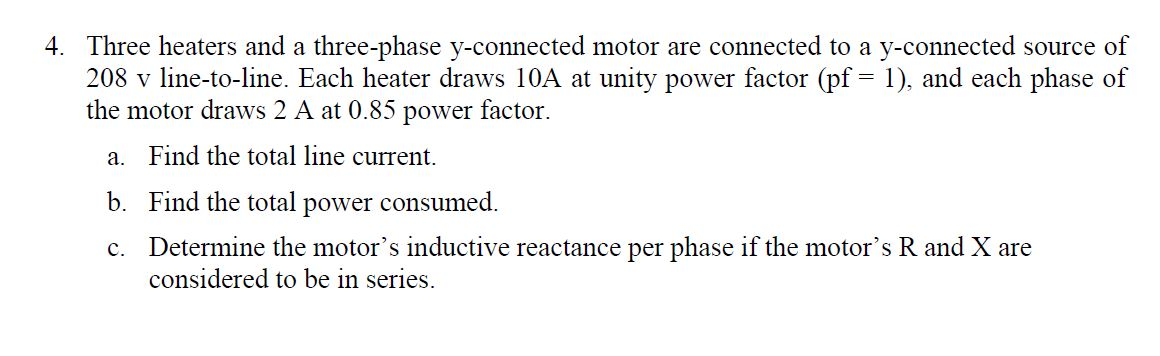# 4. Three heaters and a three-phase y-connected motor are connected to a y-connected source of 208 v line-to-line. Each heater draws 10A at unity power factor (pf 1), and each phase of the motor draws 2 A at 0.85 power factor a. Find the total line current b. Find the total power consumed. Determine the motor's inductive reactance per phase if the motor's R and X are considered to be in series C.

Question

The photo below has the question. Thanks!!help_outlineImage Transcriptionclose4. Three heaters and a three-phase y-connected motor are connected to a y-connected source of 208 v line-to-line. Each heater draws 10A at unity power factor (pf 1), and each phase of the motor draws 2 A at 0.85 power factor a. Find the total line current b. Find the total power consumed. Determine the motor's inductive reactance per phase if the motor's R and X are considered to be in series C. fullscreen

### Want to see the step-by-step answer?